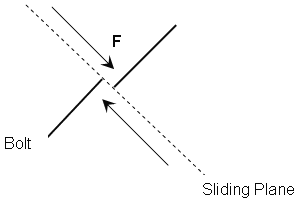The Rocscience International Conference 2021 Proceedings are now available. Read Now

# Bolt Shear Strength

The Shear Strength of a bolt can be considered in the analysis for any of the bolt types in RocPlane except the Simple Bolt Force model.

To consider bolt Shear Strength:

1. Select the Use Shear Strength check box for a Bolt Property in the Bolt Properties dialog.
2. Enter a value of shear strength. The shear strength is simply entered as a force.

The Shear Strength of a bolt refers to the shear capacity perpendicular to the axis of the bolt (i.e., what shear force would be required to shear through the cross-section of the bolt), as shown in the figure below.Shear force F required to fail bolt

## Bolt Shear Strength Implementation

If Bolt Shear Strength is considered, it is implemented in the analysis as described in Bolt Support Force in RocPlane.

• The shear force is applied only if a bolt intersects a shearing (sliding) plane of a wedge. This corresponds to bolt failure modes D, E or F (as illustrated in Bolt Support Force in RocPlane).
• Shear force is NOT applied if a bolt intersects a dilating plane (failure modes A, B, or C).
• If shear force is applied, the direction of the applied shear force is always exactly opposite to the sliding direction of the wedge.

## Bolt Efficiency and Shear Strength

Note that Bolt Orientation Efficiency is NOT used in conjunction with the Bolt Shear Strength option. If you have selected the Use Bolt Shear Strength option and the bolt failure mode is D, E or F so that the Bolt Shear Strength is applied, the Bolt Orientation Efficiency DOES NOT multiply the shear strength. The Bolt Orientation Efficiency is used only as a factor for the tensile Bolt Capacity determined from the Bolt Force Diagram. It has no effect on the Bolt Shear Strength.